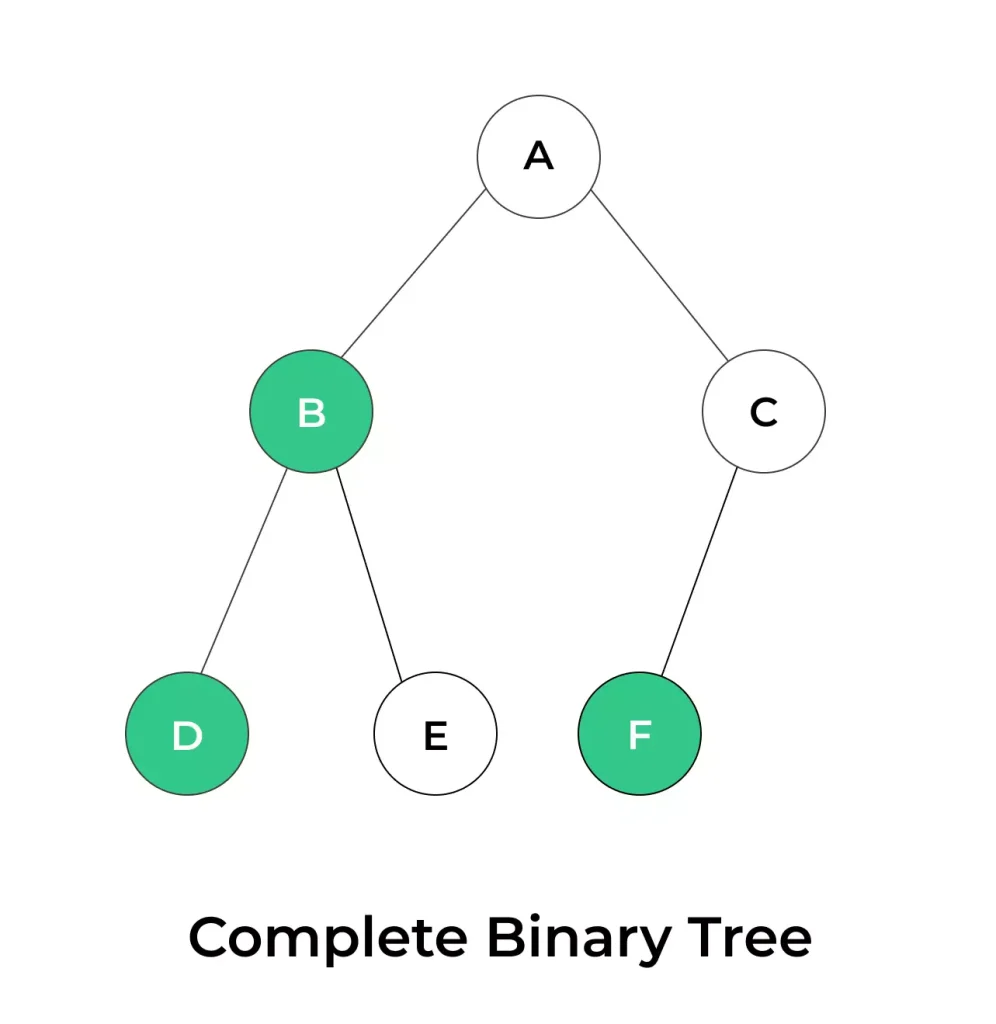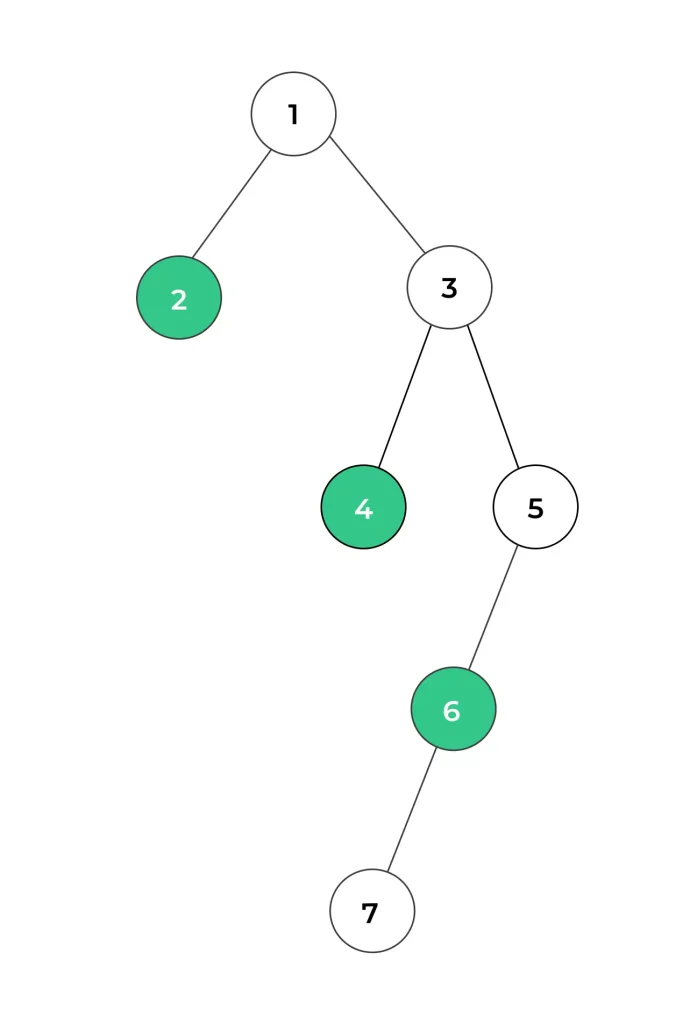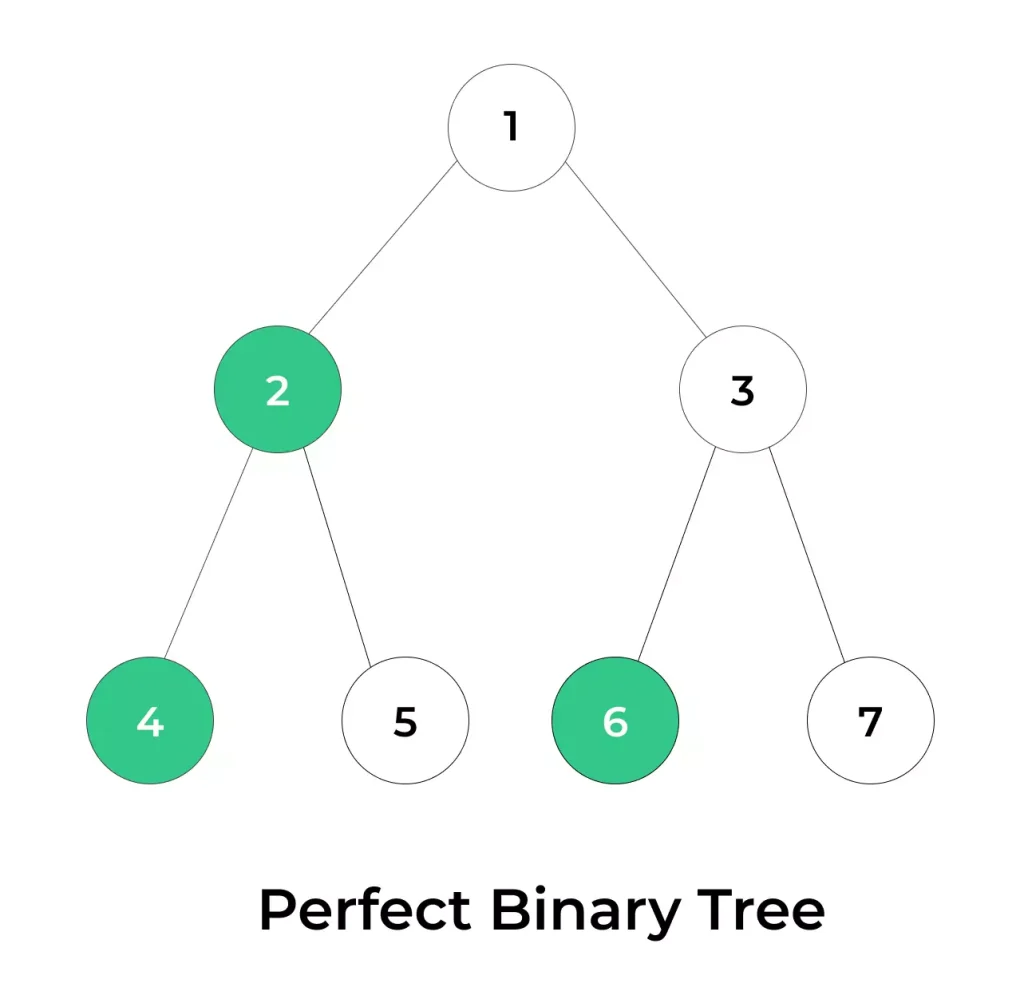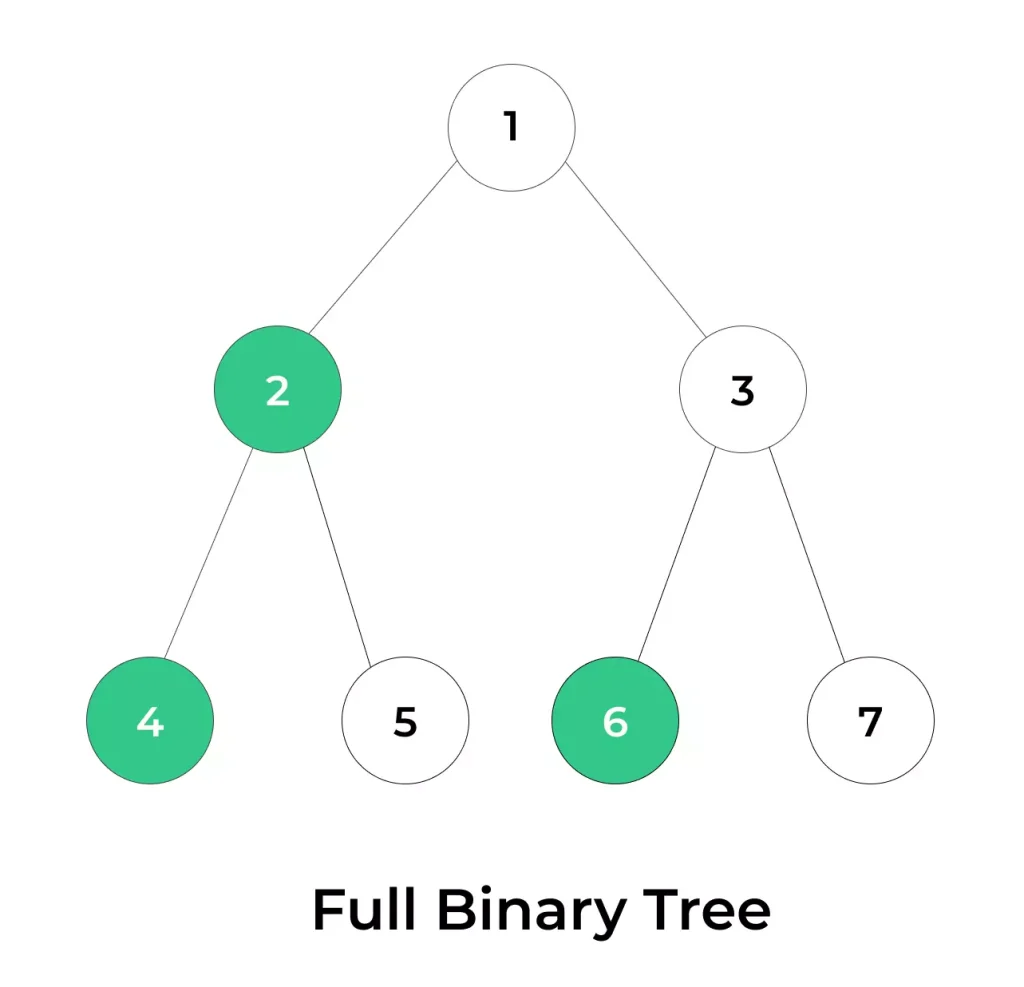# Introduction to Complete Binary Tree## Introduction to Complete Binary Tree:

A complete binary tree is a fundamental concept in binary tree structures, which plays a significant role in data structures and algorithms. This type of binary tree exhibits a specific arrangement of nodes that distinguishes it from other binary trees.

“Discover the concept of a Complete Binary Tree: a structured data tree where every level is nearly filled, ensuring efficient storage and applications in priority queues and various algorithms.”

## Complete Binary Tree:

A complete binary tree is a type of binary tree where all the levels are filled with nodes, except for the last level. In the last level, the nodes are placed from left to right without any gaps.

• Complete binary trees are used in computer science because they are efficient to work with and can be easily stored in arrays, making them practical for various algorithms and data structures like heaps and priority queues.### Here are the key characteristics of a complete binary tree:

1. All levels of the tree are completely filled, except the last level.

2. In the last level, all nodes are positioned as far to the left as possible. This means that there are no “gaps” between nodes in the last level, and nodes are filled from left to right.

3. The height of the tree is minimized for the given number of nodes. In other words, the tree is as balanced as possible.

## Creation of Complete Binary Tree:

1. Start with an Empty Tree: Begin with an empty binary tree. This tree has no nodes initially.

2. Add Nodes from Left to Right: To maintain the completeness property, you should add nodes to the tree from left to right in a level-order manner. Level-order means that you fill one level completely before moving to the next level. You start with the root node, and then for each subsequent node, you move to the left child first, and if the left child is already present, you move to the right child.

3. Repeat Step 2: Continue adding nodes in this manner until you’ve added all the desired nodes to the tree. Be sure to follow the left-to-right order for each level.

4. Completing the Last Level: In most cases, the last level of a complete binary tree may not be completely filled. To deal with this, add nodes from left to right, skipping any gaps, until you’ve added all the nodes for that level.

5. Tree Is Now Complete: Once you’ve added all the nodes following the left-to-right pattern, your binary tree will be complete.## Example of Complete Binary Tree:

1. In this example, nodes are added in a level-order manner, and the last level is filled from left to right, even if there are gaps.
2. Remember that the order in which nodes are added to the tree is crucial to maintain the completeness property of the tree.
3. This process ensures that the tree remains balanced and can be efficiently used in various algorithms and data structures.

## Perfect Binary Tree vs Complete Binary Tree

Property Perfect Binary TreeComplete Binary Tree
Definition: A binary tree in which all levels are completely filled with nodes.A binary tree in which all levels are completely filled, except possibly for the last level, which is filled from left to right.
Shape: Balanced and symmetrical.Not necessarily balanced; can have an unbalanced shape.
Use Cases: Less common in practice, more theoretical.More common and practical in various applications.
Height: Log₂(n + 1) where ‘n’ is the number of nodes.Can vary; height depends on the number of nodes and their arrangement.
No of Nodes: 2^n – 1, where ‘n’ is the level of the tree.Can have between 2^(h-1) and 2^h – 1 nodes, where ‘h’ is the height of the tree.## Full Binary Tree vs Complete Binary Tree

Property Full Binary Tree Complete Binary Tree
Definition: Every node has 0 or 2 children.All levels are filled except possibly the last level. In the last level, nodes are positioned from left to right.
Structure: Highly structured and rigid.Flexible structure with some constraints. All nodes are positioned as far left as possible in the last level.
Balanced Structure: Perfectly balanced.Relatively balanced, but not as strictly as a full binary tree.
Height: Height ‘h’ is ‘h’.Height ‘h’ is minimized for the given number of nodes.
No of Nodes: 2^h – 1 (for height ‘h’).Between 2^h and 2^(h+1) – 1 (for height ‘h’).• A full binary tree, also known as a proper binary tree or a 2-tree, is a type of binary tree in which each node has either 0 or 2 children.
• In this example, every non-leaf node (1, 2, and 3) has exactly two child nodes, and the leaf nodes (4, 5, 6, and 7) have no children. This tree adheres to the definition of a full binary tree because it maintains the property of having either 0 or 2 children for each node throughout the entire structure.

#### key terminologies of Complete binary tree

1. Root: The topmost node in the tree, from which all other nodes are descended.

2. Node: A data element in the tree, consisting of a value and references to its child nodes.

3. Parent: A node that has one or more child nodes connected below it.

4. Child: A node directly connected to another node below it in the hierarchy.

5. Leaf Node: A node with no child nodes; it is located at the bottom level of the tree.

6. Height: The length of the longest path from the root node to a leaf node in the tree. The height of a complete binary tree is typically defined by the number of levels.

7. Level: The layers or depths of nodes in the tree, starting with level 0 at the root and increasing as you move away from the root.

### Related Banners

Get PrepInsta Prime & get Access to all 200+ courses offered by PrepInsta in One Subscription

## Get over 200+ course One Subscription

Courses like AI/ML, Cloud Computing, Ethical Hacking, C, C++, Java, Python, DSA (All Languages), Competitive Coding (All Languages), TCS, Infosys, Wipro, Amazon, DBMS, SQL and others